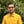Trusted answers to developer questions
Trusted Answers to Developer Questions

Related Tags

ruby
math
communitycreator

# What is Math.tan() in Ruby?Harris Amjad

Grokking Modern System Design Interview for Engineers & Managers

Ace your System Design Interview and take your career to the next level. Learn to handle the design of applications like Netflix, Quora, Facebook, Uber, and many more in a 45-min interview. Learn the RESHADED framework for architecting web-scale applications by determining requirements, constraints, and assumptions before diving into a step-by-step design process.

The tan() function calculates and returns the tangent of a number. To be more specific, it returns the tangent of a number in radians.

Figure 1 shows the mathematical representation of the tan() function.

Figure 1: Mathematical representation of tangent function

Note:

• This function is present in the Math module.
• This tan() function only works for right-angled triangles.

## Syntax

tan(num)


## Parameter

This function requires a number that represents an angle in radians as a parameter.

To convert degrees to radians, use the following formula.

radians = degrees * ( pi / 180.0 )


## Return value

tan() returns the tangent of a number that is set as a parameter. The return value is in the range [-1,1].

## Example

#positive number in radiansputs "The value of tan(2.3) : ",  Math.tan(2.3)#negative number in radiansputs "The value of tan(-2.3) : ",  Math.tan(-2.3)#converting the degrees angle into radians and then applying tan()#degrees = 45#PI = 3.14159265result = 45 * (Math::PI / 180.0)puts "The value of tan(#{result}) : ",  Math.tan(result)

RELATED TAGS

ruby
math
communitycreator

CONTRIBUTORHarris Amjad

Grokking Modern System Design Interview for Engineers & Managers

Ace your System Design Interview and take your career to the next level. Learn to handle the design of applications like Netflix, Quora, Facebook, Uber, and many more in a 45-min interview. Learn the RESHADED framework for architecting web-scale applications by determining requirements, constraints, and assumptions before diving into a step-by-step design process.

Keep Exploring

Learn in-demand tech skills in half the time